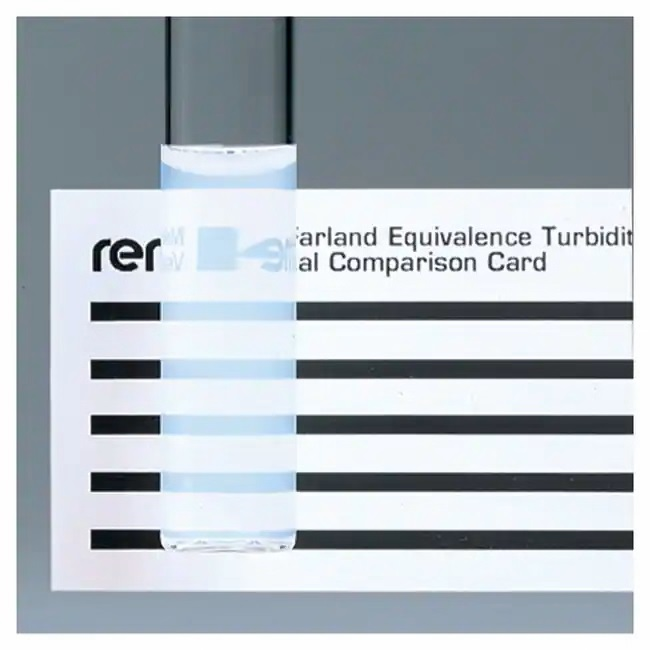## Remel™ McFarland Equivalence Turbidity Standards

Remel McFarland Equivalence Turbidity Standards are used as standards in adjusting densities of bacterial suspensions.

• R20410, McFarland Equivalence Turbidity Standard 0.5 .Each
• R20411, McFarland Equivalence Turbidity Standard 1.0 . Each
• R20412, McFarland Equivalence Turbidity Standard 2.0 .Each
• R20413, McFarland Equivalence Turbidity Standard 3.0 .Each
• R20414, McFarland Equivalence Turbidity Standard 4.0.. Each
• R20415, McFarland Equivalence Turbidity Standard 5.0. Each
• R20421, McFarland Equivalence Turbidity Standard Set..Set* *Contains 1 each of 0.5, 1.0, 2.0, 3.0, and 4.0 standards

•• Tube size is 15 x 103mm and fits in most spectrophotometers
• Polystyrene technology allows longer shelf life